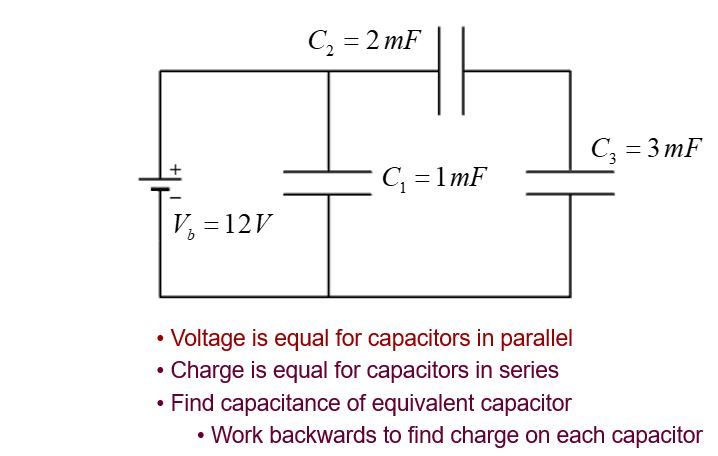﻿ Capacitors

# Capacitors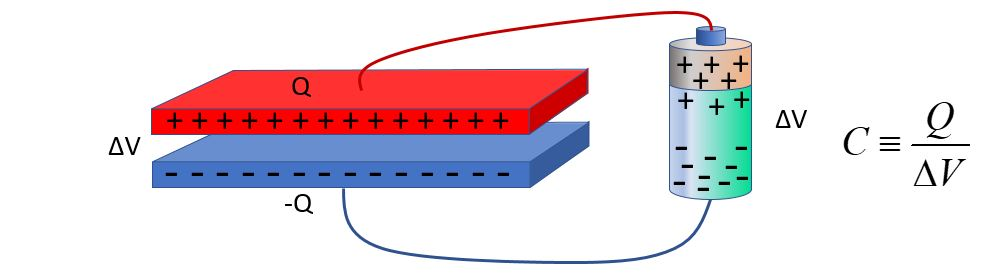### Work is done inside a battery to separate charges. This work is stored as potential energy inside the battery. When a battery is connected via wire to a capacitor, the charges flow through the wire to the capacitor until the potential difference is the same across the capacitor and the battery, if they are connected simply, as in this illustration. This assumes there is no resistance in the wire, which would use some of the energy of the electrons as they move through the wire. The capacitance, C, of the capacitor is defined as the total charge on one plate, divided by the potential difference of the capacitor. The unit for capacitance is the farad, F, equivalent to 1 C/V.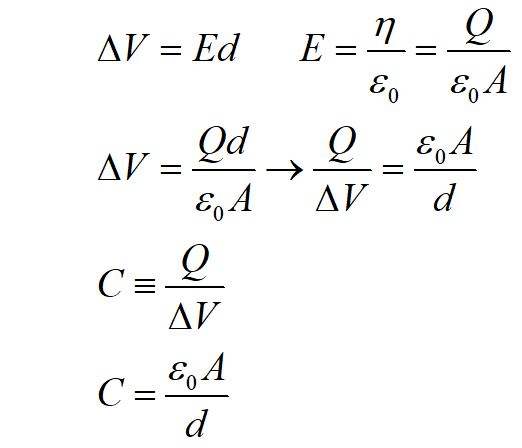### We can use the relationship between potential difference and the electrid field, along with the E field of an infinite plane, to write and expression for capacitance. The capacitance is defined as Q/ΔV, but it can be very useful to write it in terms of the physical geometry of a parallel plate capacitor.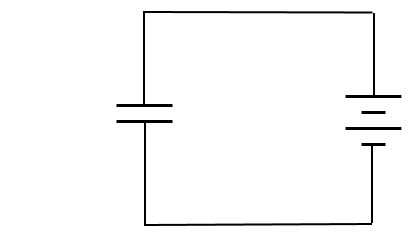### We often use diagrams to represent a configuration like that of a simple circuit like this. Here, the battery is represented by alternating long and short lines. The side with the long line represents the positive side of the battery. A capacitor is represented by two lines with equal length. The capacitor and battery are called elements of the circuit. The wire in the circuit is represented by the connecting lines. Charge flows through the wire until the potential difference of the capcitor is the same as that of the battery.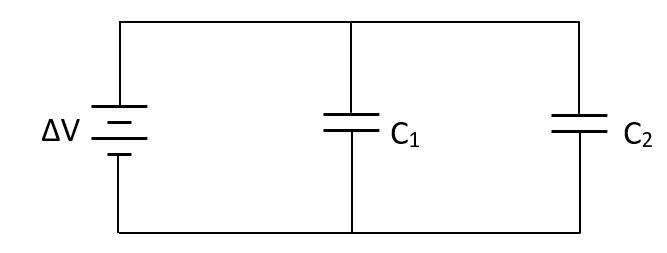### A circuit can contain more than one capacitor. These capacitors are connected in parallel. Charge flows through the wire until the capacitors are completely charged. The wire connecting the top plates of the capacitors insures that the top plates are at the same potential as the positive side of the battery. Similarly, the bottom plates are at the same potential as the negative side of the battery. Capacitors in parallel have the same potential difference.### The total charge flowing onto the parallel capacitors equals the sum of the charges of the two capacitors. We can use this, plus the fact that they have the same potential difference, to find an expression for the capacitance of the system of parallel capacitors.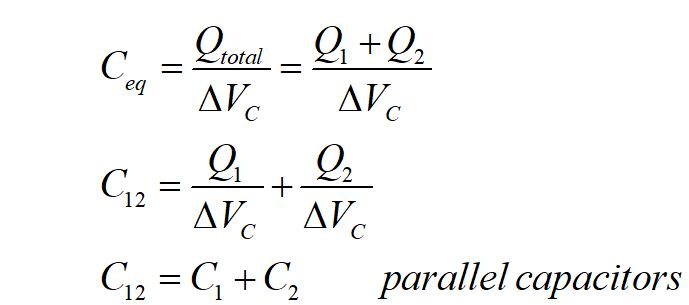### The equivalent capacitance of the two parallel capacitors is shown to be just the sum of the capacitance of the two capacitors.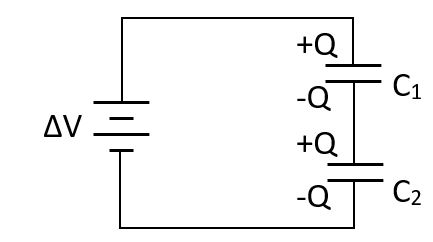### These two capacitors are connected in series. When the capacitors are fully charged, the top plate of C1 has the same potential difference of the positive side of the battery and the bottom plate of C2 has the same potential as the negative side of the battery. The segment between the capacitors is electrically isolated, so it must stay at zero total charge. The charged capacitor plates induce a charge on their opposite plates, polarizing this isolated segment, such that the individual capacitors have equal and opposite charge on their plates.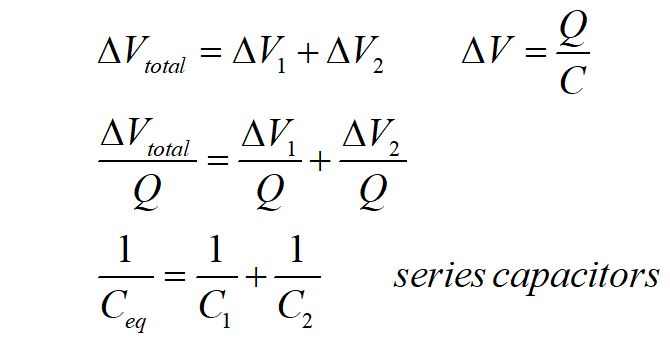### For capacitors in series, we can use the facts that their charges are equal and that their potential differences must add up to the potential difference of the battery to find an expression for equivalent capacitance of the system.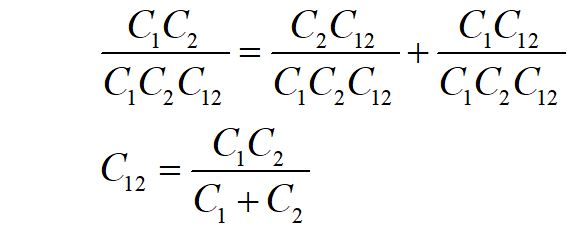## Sample questions

### 1. Consider this circuit to choose the correct statements.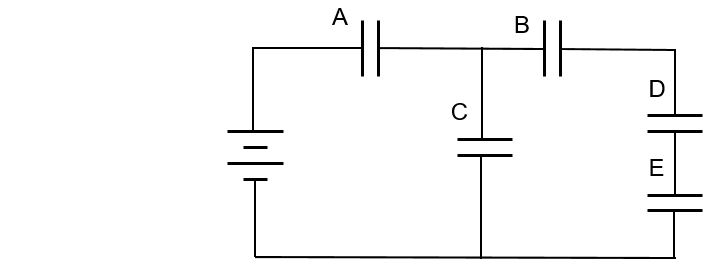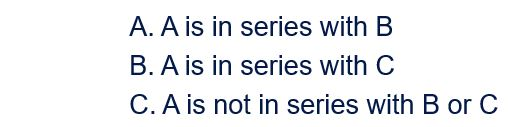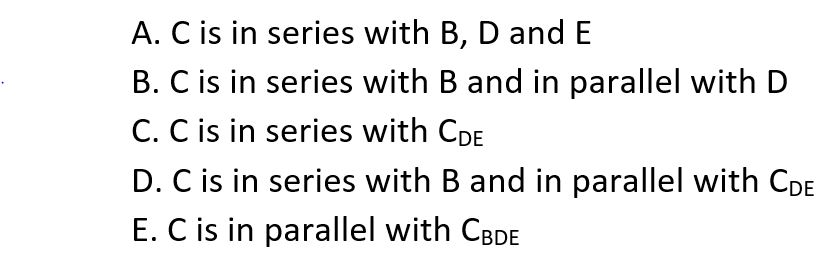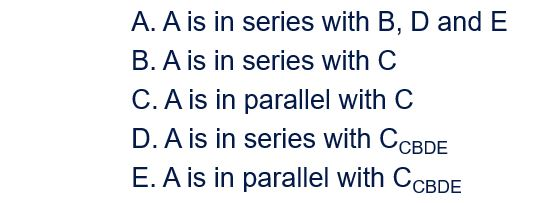### 2. Find the equivalent capacitance for this system of capacitors.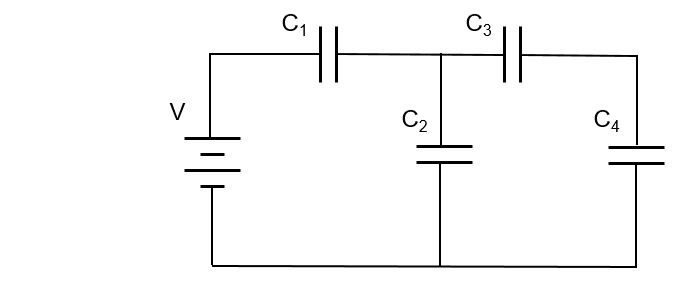## 3. Find the charge on each capacitor.